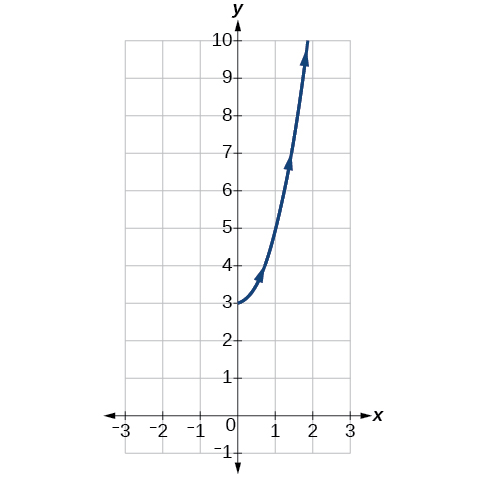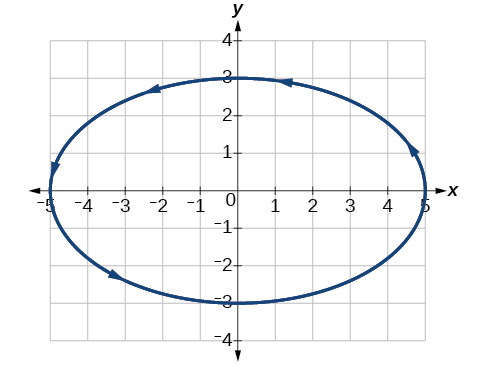# 8.7 Parametric equations: graphs

 Page 1 / 4
In this section you will:
• Graph plane curves described by parametric equations by plotting points.
• Graph parametric equations.

It is the bottom of the ninth inning, with two outs and two men on base. The home team is losing by two runs. The batter swings and hits the baseball at 140 feet per second and at an angle of approximately $\text{\hspace{0.17em}}45°\text{\hspace{0.17em}}$ to the horizontal. How far will the ball travel? Will it clear the fence for a game-winning home run? The outcome may depend partly on other factors (for example, the wind), but mathematicians can model the path of a projectile and predict approximately how far it will travel using parametric equations . In this section, we’ll discuss parametric equations and some common applications, such as projectile motion problems.Parametric equations can model the path of a projectile. (credit: Paul Kreher, Flickr)

## Graphing parametric equations by plotting points

In lieu of a graphing calculator or a computer graphing program, plotting points to represent the graph of an equation is the standard method. As long as we are careful in calculating the values, point-plotting is highly dependable.

Given a pair of parametric equations, sketch a graph by plotting points.

1. Construct a table with three columns: $\text{\hspace{0.17em}}t,x\left(t\right),\text{and}\text{\hspace{0.17em}}\text{\hspace{0.17em}}y\left(t\right).$
2. Evaluate $x$ and $y$ for values of $t$ over the interval for which the functions are defined.
3. Plot the resulting pairs $\text{\hspace{0.17em}}\left(x,y\right).$

## Sketching the graph of a pair of parametric equations by plotting points

Sketch the graph of the parametric equations $x\left(t\right)={t}^{2}+1,\text{\hspace{0.17em}}\text{\hspace{0.17em}}y\left(t\right)=2+t.$

Construct a table of values for $\text{\hspace{0.17em}}t,x\left(t\right),\text{\hspace{0.17em}}$ and $\text{\hspace{0.17em}}y\left(t\right),\text{\hspace{0.17em}}$ as in [link] , and plot the points in a plane.

$t$ $x\left(t\right)={t}^{2}+1$ $y\left(t\right)=2+t$
$-5$ $26$ $-3$
$-4$ $17$ $-2$
$-3$ $10$ $-1$
$-2$ $5$ $0$
$-1$ $2$ $1$
$0$ $1$ $2$
$1$ $2$ $3$
$2$ $5$ $4$
$3$ $10$ $5$
$4$ $17$ $6$
$5$ $26$ $7$

The graph is a parabola with vertex at the point $\text{\hspace{0.17em}}\left(1,2\right),$ opening to the right. See [link] .

Sketch the graph of the parametric equations $\text{\hspace{0.17em}}x=\sqrt{t},\text{\hspace{0.17em}}\text{\hspace{0.17em}}y=2t+3,\text{\hspace{0.17em}}\text{\hspace{0.17em}}\text{\hspace{0.17em}}0\le t\le 3.$## Sketching the graph of trigonometric parametric equations

Construct a table of values for the given parametric equations and sketch the graph:

$\begin{array}{l}\\ \begin{array}{l}x=2\mathrm{cos}\text{\hspace{0.17em}}t\hfill \\ y=4\mathrm{sin}\text{\hspace{0.17em}}t\hfill \end{array}\end{array}$

Construct a table like that in [link] using angle measure in radians as inputs for $\text{\hspace{0.17em}}t,\text{\hspace{0.17em}}$ and evaluating $\text{\hspace{0.17em}}x\text{\hspace{0.17em}}$ and $\text{\hspace{0.17em}}y.\text{\hspace{0.17em}}$ Using angles with known sine and cosine values for $\text{\hspace{0.17em}}t\text{\hspace{0.17em}}$ makes calculations easier.

$t$ $x=2\mathrm{cos}\text{\hspace{0.17em}}t$ $y=4\mathrm{sin}\text{\hspace{0.17em}}t$
0 $x=2\mathrm{cos}\left(0\right)=2$ $y=4\mathrm{sin}\left(0\right)=0$
$\frac{\pi }{6}$ $x=2\mathrm{cos}\left(\frac{\pi }{6}\right)=\sqrt{3}$ $y=4\mathrm{sin}\left(\frac{\pi }{6}\right)=2$
$\frac{\pi }{3}$ $x=2\mathrm{cos}\left(\frac{\pi }{3}\right)=1$ $y=4\mathrm{sin}\left(\frac{\pi }{3}\right)=2\sqrt{3}$
$\frac{\pi }{2}$ $x=2\mathrm{cos}\left(\frac{\pi }{2}\right)=0$ $y=4\mathrm{sin}\left(\frac{\pi }{2}\right)=4$
$\frac{2\pi }{3}$ $x=2\mathrm{cos}\left(\frac{2\pi }{3}\right)=-1$ $y=4\mathrm{sin}\left(\frac{2\pi }{3}\right)=2\sqrt{3}$
$\frac{5\pi }{6}$ $x=2\mathrm{cos}\left(\frac{5\pi }{6}\right)=-\sqrt{3}$ $y=4\mathrm{sin}\left(\frac{5\pi }{6}\right)=2$
$\pi$ $x=2\mathrm{cos}\left(\pi \right)=-2$ $y=4\mathrm{sin}\left(\pi \right)=0$
$\frac{7\pi }{6}$ $x=2\mathrm{cos}\left(\frac{7\pi }{6}\right)=-\sqrt{3}$ $y=4\mathrm{sin}\left(\frac{7\pi }{6}\right)=-2$
$\frac{4\pi }{3}$ $x=2\mathrm{cos}\left(\frac{4\pi }{3}\right)=-1$ $y=4\mathrm{sin}\left(\frac{4\pi }{3}\right)=-2\sqrt{3}$
$\frac{3\pi }{2}$ $x=2\mathrm{cos}\left(\frac{3\pi }{2}\right)=0$ $y=4\mathrm{sin}\left(\frac{3\pi }{2}\right)=-4$
$\frac{5\pi }{3}$ $x=2\mathrm{cos}\left(\frac{5\pi }{3}\right)=1$ $y=4\mathrm{sin}\left(\frac{5\pi }{3}\right)=-2\sqrt{3}$
$\frac{11\pi }{6}$ $x=2\mathrm{cos}\left(\frac{11\pi }{6}\right)=\sqrt{3}$ $y=4\mathrm{sin}\left(\frac{11\pi }{6}\right)=-2$
$2\pi$ $x=2\mathrm{cos}\left(2\pi \right)=2$ $y=4\mathrm{sin}\left(2\pi \right)=0$

By the symmetry shown in the values of $x$ and $\text{\hspace{0.17em}}y,\text{\hspace{0.17em}}$ we see that the parametric equations represent an ellipse . The ellipse is mapped in a counterclockwise direction as shown by the arrows indicating increasing $\text{\hspace{0.17em}}t\text{\hspace{0.17em}}$ values.

Graph the parametric equations: $\text{\hspace{0.17em}}x=5\mathrm{cos}\text{\hspace{0.17em}}t,\text{\hspace{0.17em}}\text{\hspace{0.17em}}y=3\mathrm{sin}\text{\hspace{0.17em}}t.$## Graphing parametric equations and rectangular form together

Graph the parametric equations $\text{\hspace{0.17em}}x=5\mathrm{cos}\text{\hspace{0.17em}}t\text{\hspace{0.17em}}$ and $\text{\hspace{0.17em}}y=2\mathrm{sin}\text{\hspace{0.17em}}t.\text{\hspace{0.17em}}$ First, construct the graph using data points generated from the parametric form . Then graph the rectangular form of the equation. Compare the two graphs.

Construct a table of values like that in [link] .

$t$ $x=5\mathrm{cos}\text{\hspace{0.17em}}t$ $y=2\mathrm{sin}\text{\hspace{0.17em}}t$
$\text{0}$ $x=5\mathrm{cos}\left(0\right)=5$ $y=2\mathrm{sin}\left(0\right)=0$
$\text{1}$ $x=5\mathrm{cos}\left(1\right)\approx 2.7$ $y=2\mathrm{sin}\left(1\right)\approx 1.7$
$\text{2}$ $x=5\mathrm{cos}\left(2\right)\approx -2.1$ $y=2\mathrm{sin}\left(2\right)\approx 1.8$
$\text{3}$ $x=5\mathrm{cos}\left(3\right)\approx -4.95$ $y=2\mathrm{sin}\left(3\right)\approx 0.28$
$\text{4}$ $x=5\mathrm{cos}\left(4\right)\approx -3.3$ $y=2\mathrm{sin}\left(4\right)\approx -1.5$
$\text{5}$ $x=5\mathrm{cos}\left(5\right)\approx 1.4$ $y=2\mathrm{sin}\left(5\right)\approx -1.9$
$-1$ $x=5\mathrm{cos}\left(-1\right)\approx 2.7$ $y=2\mathrm{sin}\left(-1\right)\approx -1.7$
$-2$ $x=5\mathrm{cos}\left(-2\right)\approx -2.1$ $y=2\mathrm{sin}\left(-2\right)\approx -1.8$
$-3$ $x=5\mathrm{cos}\left(-3\right)\approx -4.95$ $y=2\mathrm{sin}\left(-3\right)\approx -0.28$
$-4$ $x=5\mathrm{cos}\left(-4\right)\approx -3.3$ $y=2\mathrm{sin}\left(-4\right)\approx 1.5$
$-5$ $x=5\mathrm{cos}\left(-5\right)\approx 1.4$ $y=2\mathrm{sin}\left(-5\right)\approx 1.9$

Plot the $\text{\hspace{0.17em}}\left(x,y\right)\text{\hspace{0.17em}}$ values from the table. See [link] .

Next, translate the parametric equations to rectangular form. To do this, we solve for $\text{\hspace{0.17em}}t\text{\hspace{0.17em}}$ in either $\text{\hspace{0.17em}}x\left(t\right)\text{\hspace{0.17em}}$ or $\text{\hspace{0.17em}}y\left(t\right),\text{\hspace{0.17em}}$ and then substitute the expression for $\text{\hspace{0.17em}}t\text{\hspace{0.17em}}$ in the other equation. The result will be a function $y\left(x\right)$ if solving for $\text{\hspace{0.17em}}t\text{\hspace{0.17em}}$ as a function of $\text{\hspace{0.17em}}x,\text{\hspace{0.17em}}$ or $\text{\hspace{0.17em}}x\left(y\right)$ if solving for $\text{\hspace{0.17em}}t\text{\hspace{0.17em}}$ as a function of $\text{\hspace{0.17em}}y.\text{\hspace{0.17em}}$

Then, use the Pythagorean Theorem .

$\begin{array}{r}\hfill {\mathrm{cos}}^{2}t+{\mathrm{sin}}^{2}t=1\\ \hfill {\left(\frac{x}{5}\right)}^{2}+{\left(\frac{y}{2}\right)}^{2}=1\\ \hfill \frac{{x}^{2}}{25}+\frac{{y}^{2}}{4}=1\end{array}$

a colony of bacteria is growing exponentially doubling in size every 100 minutes. how much minutes will it take for the colony of bacteria to triple in size
I got 300 minutes. is it right?
Patience
no. should be about 150 minutes.
Jason
It should be 158.5 minutes.
Mr
ok, thanks
Patience
what is the importance knowing the graph of circular functions?
can get some help basic precalculus
What do you need help with?
Andrew
how to convert general to standard form with not perfect trinomial
can get some help inverse function
ismail
Rectangle coordinate
how to find for x
it depends on the equation
Robert
whats a domain
The domain of a function is the set of all input on which the function is defined. For example all real numbers are the Domain of any Polynomial function.
Spiro
foci (–7,–17) and (–7,17), the absolute value of the differenceof the distances of any point from the foci is 24.
difference between calculus and pre calculus?
give me an example of a problem so that I can practice answering
x³+y³+z³=42
Robert
dont forget the cube in each variable ;)
Robert
of she solves that, well ... then she has a lot of computational force under her command ....
Walter
what is a function?
I want to learn about the law of exponent
explain this
what is functions?
A mathematical relation such that every input has only one out.
Spiro
yes..it is a relationo of orders pairs of sets one or more input that leads to a exactly one output.
Mubita
Is a rule that assigns to each element X in a set A exactly one element, called F(x), in a set B.
RichieRich
If the plane intersects the cone (either above or below) horizontally, what figure will be created?

#### Get Jobilize Job Search Mobile App in your pocket Now!ByBy OpenStaxBy Tod McGrathBy Marion CabalfinBy Jonathan LongBy Zarina ChocolateBy RhodesBy Jonathan LongBy OpenStaxBy OpenStaxBy OpenStax